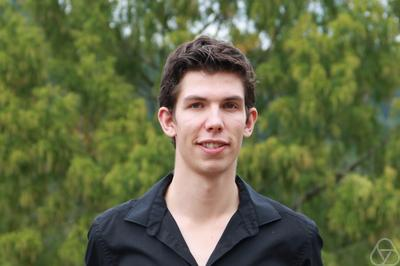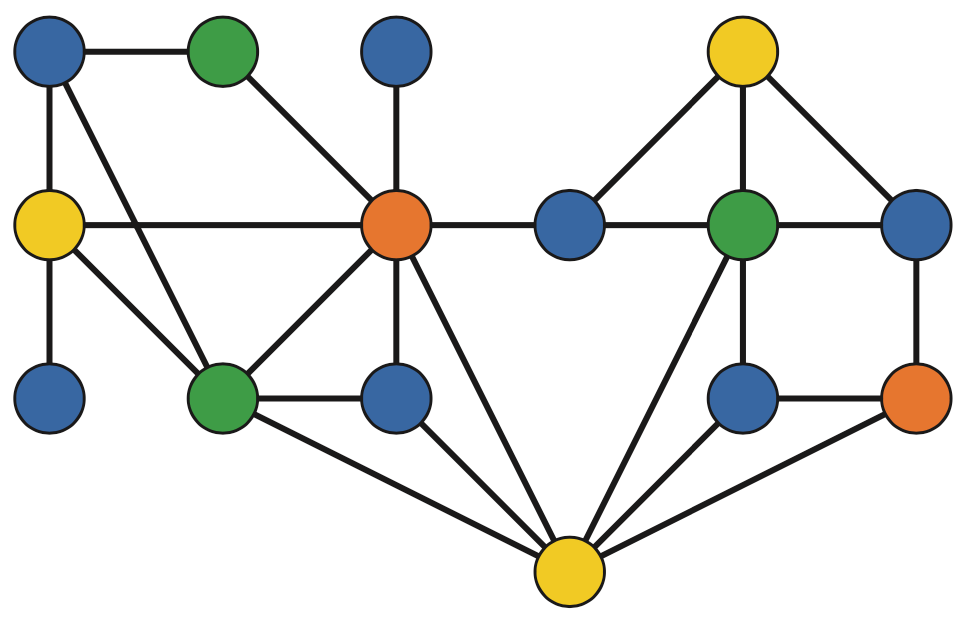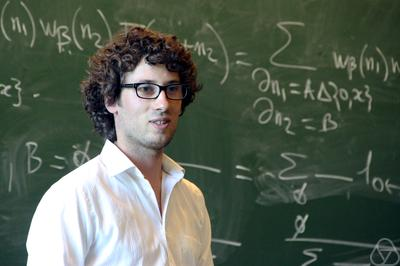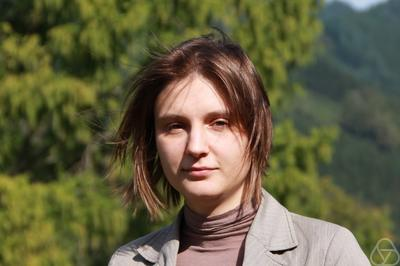# 2022 Fields medal winners

Albert Wood returns to introduce the work that has won mathematics’ most famous award this year.When I tell people that I work in mathematics research, I’m often met with a baffled expression. “You can research mathematics? I thought it was already all figured out!” It’s hard enough to explain my own work; you’d be forgiven for thinking that the winners of the Fields medal, the most famous of mathematical achievements, must work on the most incomprehensible and inapplicable mathematics of all.The 2022 Fields medals were awarded at Aalto University in Helskinki. Image: Wikimedia commons user KFP, CC BY-SA 3.0.

In fact, nothing could be further from the truth. The work of 2022 Fields medal winners James Maynard, June Huh, Maryna Viazovska and Hugo Duminil-Copin shows how modern mathematical techniques can be applied to break open age-old problems, of the kind that anyone can appreciate. Next time I’m asked what mathematics research is about, I will refer to the recipients of the most prestigious maths award in the world for the answer: ingenious solutions to beautiful questions.

## James MaynardJames Maynard. Image: Mathematisches Forschungsinstitut Oberwolfach, CC BY-SA 2.0 DE

The very first Fields medal was given at the International Congress of Mathematicians (ICM) in 1936, although the congress itself dates back even further. In fact, it was at the 1912 ICM that Edmund Landau famously stated his four ‘unattackable’ conjectures, which have inspired generations of number theorists, and remain unsolved to this day:

• There are infinitely many pairs of primes with a difference of 2 (the twin prime conjecture)
• Every even integer is the sum of two primes (the Goldbach conjecture)
• There is a prime number between any two consecutive squares
• There are infinitely many primes of the form $n^2 + 1$.

James Maynard works in the field of analytic number theory, which aims to resolve questions like these by estimating the quantity of numbers satisfying these criteria, rather than working with the numbers themselves. In 2016, he proved that there are infinitely many prime numbers that do not contain the digit 7.

When you think about it, this result is truly mindblowing. Since extremely large numbers should contain an extremely large number of 7s (the largest known prime number contains over 2 million!), the probability of containing none at all is absolutely minute.

Among analytic number theorists, he is most well known for his ‘primes in bounded gaps’ theorem. He was able to show that, for some width $W$, there are infinitely many pairs of primes with a difference of at most $W$ and if we want to find sets of 3, or 4, or $m$ primes instead of pairs, there’s always a $W$ that will give us infinitely many. Although this doesn’t quite imply the famous twin prime conjecture, it is very much in that spirit and the conjecture is firmly in his sights.

## June HuhJune Huh. Image: Mathematisches Forschungsinstitut Oberwolfach, CC BY-SA 2.0 DE

Given four points on a plane, how many different ways are there to draw a straight line through at least two of them? A quick sketch on paper may suggest that the answer is six, but in fact it depends on the positions of the points: if three are in a row then the answer is four lines, and if they all line up then there is only one.

The question of how many lines may be drawn in general is one of combinatorics: the maths of counting and the specialist subject of June Huh, the first Korean recipient of the Fields medal. One of his most important results tackles the generalisation of the lines and dots problem to higher dimensions.

When we put our points in $n$-dimensional space, we can ask how many lines, planes, and $d$-dimensional hyperplanes they determine. Although the numbers depend on how the points are arranged, June showed that the sequence of numbers displays a pattern known as unimodality: as $d$ increases, the number of hyperplanes increases to a maximum and then decreases. No bouncing around!This graph has been coloured so that no two adjacent nodes are the same colour.

June has also made groundbreaking contributions to the field of graph theory, which examines the properties of networks of nodes connected by edges. A famous question asks for the number of ways a graph can be coloured, so that no two adjacent nodes have the same colour. To understand how these numbers behave, graph theorists use chromatic polynomials. June’s results proved that a 40-year-old conjecture about chromatic polynomials is true, including establishing that their coefficients are unimodal.

June’s most important contributions to the field of combinatorics are arguably not the results themselves, but his method of proof, which uses advanced results from the vastly different world of algebraic geometry. By forging a link between these distant fields, he brings the mathematical community closer together, and unveils hints that mathematics still has many secrets left to offer.

## Hugo Duminil-CopinHugo Duminil-Copin. Image: Mathematisches Forschungsinstitut Oberwolfach, CC BY-SA 2.0 DE

A magnet at room temperature will stick to a metal sheet. Heat the magnet up, and there will come a moment where it suddenly falls to the ground. This is a dramatic example of a phase transition in physics, but why does it happen?

In order to understand such problems, mathematicians like Hugo Duminil-Copin create mathematical models of the physical systems. In this case, one suitable model is the Ising model, which consists of a lattice of points representing the atoms, and an assignment of $1$s and $-1$s to the points representing the ‘spin’ of the nuclei. The spin of each nucleus is influenced by the spins of its neighbours; by studying the distribution of spins at a particular temperature, we can understand the properties of the magnet itself.

One key problem with these models is that their assumptions are unrealistic. For example, in real magnets the atoms aren’t arranged in perfect grids! One of Hugo’s main results is in demonstrating that phase transitions do not depend on how the atoms are arranged. This means that the assumptions are justified after all.

Hugo has also applied his techniques of statistical physics to seemingly unrelated mathematical conundrums. For example, one of his recent results helps us to count the number of ways to cross a honeycomb from left to right without ever revisiting a cell. This is an easy problem when the honeycomb is small, but extremely tricky for bigger sections. Solving major open problems considered impossible is said to be one of Hugo’s trademarks.

## Maryna ViazovskaMaryna Viazovska. Image: Mathematisches Forschungsinstitut Oberwolfach, CC BY-SA 2.0 DE

Maryna Viazovska’s most famous result is a perfect example of her unique approach. Her work is on sphere packing, which you can find in practice in any supermarket. The most space-efficient way to stack oranges is to fill the first layer, and then work upwards, with the oranges snugly fitting in the gaps from the layer below. Although greengrocers have been stacking oranges like this for centuries, it took almost 400 years to prove that this is the best way, from the initial conjecture of Johannes Kepler to the eventual proof of Thomas Hales. Even then, the proof was long, messy, and required extensive use of computers.In two-dimensional space, hexagonal packing is the most efficient. In higher dimensional space, it’s less obvious what the most efficient packing arrangement is. Image: Böhringer Friedrich, CC BY-SA 2.5

Maryna’s work is on the higher-dimensional version of the orange problem. Exceptionally space-efficient packing techniques had been discovered for stacking 8-dimensional oranges in 8-dimensional space, and 24-dimensional oranges in 24-dimensional space, known as the $E_8$ lattice and Leech lattice respectively. However, as with our 3-dimensional oranges, it wasn’t clear whether these techniques were optimal.

Researchers knew that the missing link to prove optimality was a ‘magic function’, describing how tightly it’s possible to pack the spheres. The search for this function seemed so hopeless that Thomas Hales admitted ‘I felt that it would take a Ramanujan to find it’. In 2016, this is exactly what Maryna did. She used tools from number theory, including modular forms, to construct the magic function in a way that would have certainly impressed Ramanujan himself. Her technique is all the more impressive for its simplicity, especially when compared to Hales’ lengthy computer-assisted argument.

As the second female recipient and the second Ukrainian recipient of the Fields medal, Maryna is a fantastic ambassador for the global nature of mathematics in the 21st century.Albert Wood is a postdoctoral researcher working with geometric flows: physics-inspired motion of curves, surfaces, and their higher-dimensional generalisations. His quest for mathematical truth has most recently taken him to Taiwan, where he takes breaks from research by cycling up nearby volcanoes.